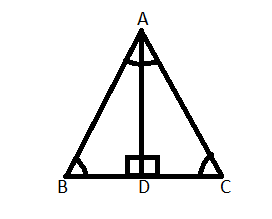QUESTION

# Prove that:Angles opposite to equal sides of an isosceles triangle are equal.

Hint: In order to solve this problem draw a triangle whose two sides are equal and then drop the perpendicular from the angle which is made by two equal sides and prove triangles congruent. Doing this will solve this problem.

The diagram for this problem can be drawn as:It is an isosceles triangle whose two sides are equal. Here those sides are AB and AC.
So, AB = AC ………(1)
We have dropped the perpendicular from A to D so we can say that angle BAD = angle CAD. It is the property of an equilateral triangle that if a perpendicular is dropped from the angle made by the two equal sides then that angle is bisected by the perpendicular………………(2)
Now on considering triangles ABD and ACD we can say
AB = AC (From 1)
$\angle BAD = \angle CAD$……….(From 2)
So, we can say $\Delta ABD \cong \Delta ACD$ (By Side-Angle-Side congruence)
Then we can say that $\angle ABD = \angle ACD$ (Angles of congruent triangles)